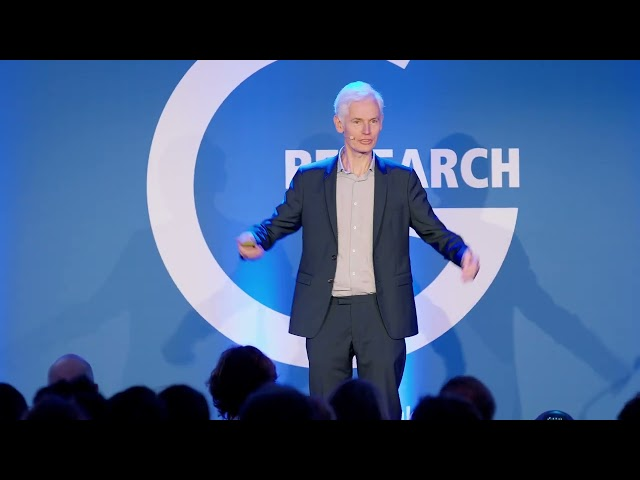# Exploring Mathematics: AI Challenges & Human Aspects | G-ResearchTLDR Mathematics involves not only well-formed statements and proofs, but also the challenges and limitations of using AI in mathematical problem-solving, and the importance of understanding the human aspects of mathematical research.

## Key insights

• 💡
Understanding the nature of mathematics is important in order to comprehend the process of human research in the field, despite the argument that humans shouldn't be able to conduct mathematical research.
• 🧩
The possibility of using AI to discover the entirety of mathematics, either from scratch or with a foundation of existing knowledge, is a fascinating and open problem.
• 🧩
The ability of computers to solve complex mathematical problems, such as those in the IMO, challenges traditional notions of human intelligence in mathematics.
• 🌳
The goal is to teach computers to choose the sensible next step in problem-solving, rather than relying on brute force searches, similar to how experienced mathematicians approach problems.
• 🤔
Researchers are interested in understanding what makes certain search problems, like finding proofs, easier for humans, in order to develop better programs and make theoretical advances in the field of mathematics.
• 📏
"What's it mean to say that the function does not tend to zero as T times infinity and this is what it means it means you can find some Epsilon." - Sir Timothy Gowers discusses the concept of a function not tending to zero as T approaches infinity.
• 💡
The question of why math is possible is an intriguing theoretical problem that deserves further exploration and insight.
• 🚀
The integration of deep learning approaches in mathematics training has the potential to revolutionize problem-solving and automate complex tasks.

## Timestamped Summary

• 📚
00:00
Mathematics is not just about well-formed statements, but also about understanding the interesting aspects of human research in mathematics, including the challenges of finding proofs and the limitations of using AI in mathematical problem-solving.
• 📚
08:54
Computers using large language models have solved 10 IMO problems, a proof involving the binomial theorem and quadratic expression is explained, and the limitations of language models in solving math problems are discussed.
• 📊
15:27
The speaker developed a program to find proofs for mathematical expressions, focusing on choosing the sensible next step rather than brute force searches, with the goal of creating a more powerful program and understanding why humans can find proofs.
• 📚
23:14
The speaker discusses a problem-solving technique called proof by negation and explains the process of moving knots and expanding the concept of a function tending to zero as t approaches infinity.
• 🧩
30:33
Finding an X with infinitely many properties in mathematics can be achieved by using the nested intervals property, which involves finding a sequence of nested intervals where each interval satisfies a specific property.
• 📝
34:44
To prove a mathematical property, it is enough to prove it for one case, and by manipulating variables, we can obtain the desired result, with a focus on the relationship between alpha and beta.
• 📚
39:36
Simplifying mathematical problems by making substitutions and choosing minimal values allows for easier solutions and progress in proving inductive hypotheses.
• 🧠
45:38
Breaking problems into smaller steps helps computer algorithms find solutions, and while deep learning is exciting, traditional AI methods are still important in finding proofs and solving unsolved math problems.Report the articleThank you for the feedback